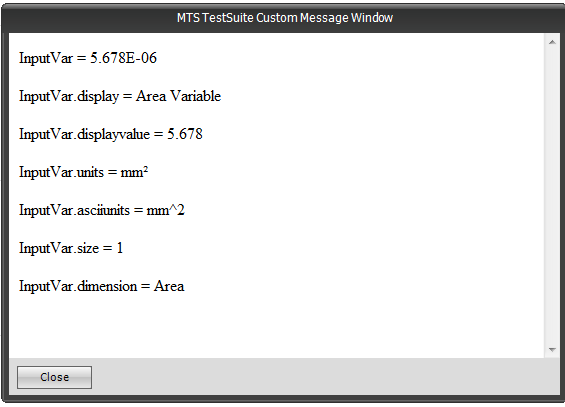# How to create calculated descriptive variable names in TestSuite

QUESTION

In TestWorks 4 you can use a formula with parameter “input1” having a value of 10 kg.   The display name can be written as Strain at {Input1} {!input1} and will appear as  “Strain at 10 kgf”  How can this be done in TestSuite TWE?

Create a text variable with the following equation:

“Strain at “ + Input1. displayvalue + “ “ +input1.units

Or

“Strain at “ + Input1. displayvalue + “ “ +input1. asciiunits

After the variable is calculated, it will contain a string that displays the desired information.

TestSuite’s variable syntax allows displaying a lot of information about a specific variable.  The following is a list of capabilities. Replace the “var” portion with the internal name of the variable.

var.display          - returns the display name of the variable

var.displayvalue  - returns the value of the variable using the display units

var.units                  - returns the display units of the variable

var.asciiunits          - returns the display units of the variable using ASCII characters. For example, millimeters raised to the second power would be returned as mm^2.

var.size                     - returns the number of values in a variable

var.dimension       - returns the dimension of the variable (Force, Length, etc.)

var.identifier         - returns the internal name of the variable

Examples below (Figure 1).Figure 1

K
Keary is the author of this solution article.

Did you find it helpful? Yes No

Send feedback